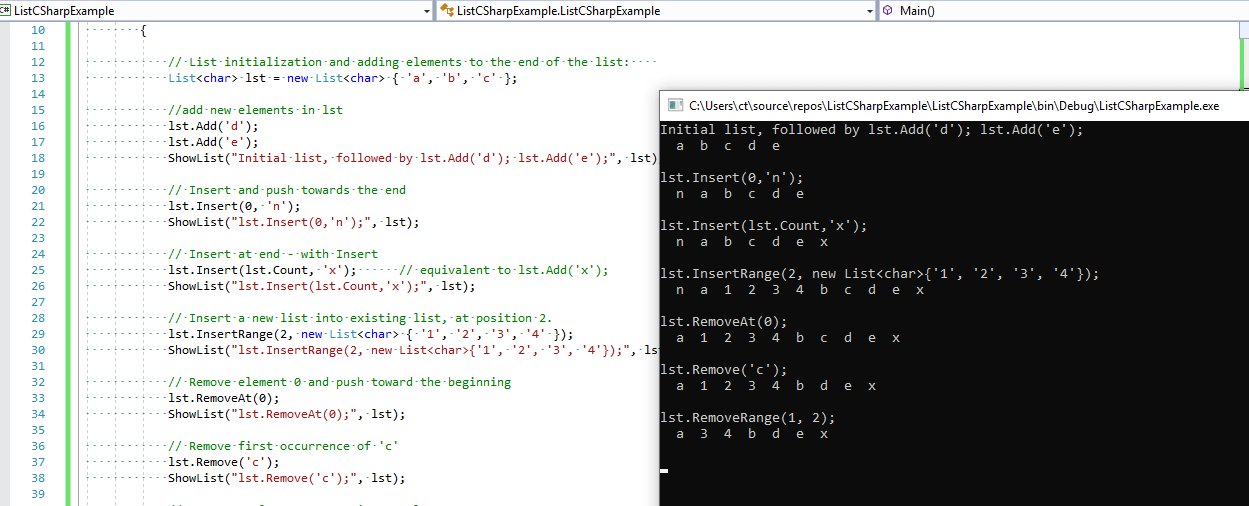# C# List

In C#, List allows us to store data of same type, means it will allow storing only strongly typed objects and size of C# list can be increased or decreased dynamically. Both `List<T>` and `Collection<T>` implement the same interface, namely `IList<T>.`

The List class gives you everything that an arraylist gives you, with the advantage that it is also typesafe. It is newer than the ArrayList class, being based on the generic features provided by a more recent version of the C# language.

### C# List Declaration

To declare the instance of list, we can have following code

``List<T> lst = new List<T>();``

in the above code, the angle (<>) brackets will indicate that the list is a generic type, where T = type parameter and it can be of any data type or class type.

### C# List Initialization

Now, let's try to initialize the list of `int` type

``List<int> lst = new List<int>();``

so the above List "lst" can have data of type "int".

Although we have many methods of C# list, but at first here I am mentioning, the method without which we cannot have data inside the list, so for adding a dat inside the list we use list.Add() method, for example

``````lst.Add(5);

### Remove Data from list

To remove data from list we have three methods, which we can call

• Remove: It is used to remove the first occurrence of a specified element from the List.
• RemoveAt: It is used to remove an element from the List based on the specified index position.
• RemoveRange: It is used to remove a range of elements from the List.

Example:

``lst.Remove(1); // remove the first occurence of '1' from int type list``

### C# List Example

Let's take a look at an C# list example, using below example, we will create list and will add/remove items from the list.

``````using System;
using System.Collections.Generic;
namespace ListCSharpExample
{

public class ListCSharpExample
{

public static void Main()
{

// List initialization and adding elements to the end of the list:
List<char> lst = new List<char> { 'a', 'b', 'c' };

// Insert and push towards the end
lst.Insert(0, 'n');
ShowList("lst.Insert(0,'n');", lst);

// Insert at end - with Insert
lst.Insert(lst.Count, 'x');      // equivalent to lst.Add('x');
ShowList("lst.Insert(lst.Count,'x');", lst);

// Insert a new list into existing list, at position 2.
lst.InsertRange(2, new List<char> { '1', '2', '3', '4' });
ShowList("lst.InsertRange(2, new List<char>{'1', '2', '3', '4'});", lst);

// Remove element 0 and push toward the beginning
lst.RemoveAt(0);
ShowList("lst.RemoveAt(0);", lst);

// Remove first occurrence of 'c'
lst.Remove('c');
ShowList("lst.Remove('c');", lst);

// Remove 2 elements, starting at element 1
lst.RemoveRange(1, 2);
ShowList("lst.RemoveRange(1, 2);", lst);

}

//loop through list using foreach
public static void ShowList<T>(string explanation, List<T> list)
{
Console.WriteLine(explanation);
foreach (T el in list)
{
Console.Write("{0, 3}", el);
}
Console.WriteLine(); Console.WriteLine();
}

}
}
``````

Output:

``````Initial list, followed by lst.Add('d'); lst.Add('e');
a  b  c  d  e

lst.Insert(0,'n');
n  a  b  c  d  e

lst.Insert(lst.Count,'x');
n  a  b  c  d  e  x

lst.InsertRange(2, new List<char>{'1', '2', '3', '4'});
n  a  1  2  3  4  b  c  d  e  x

lst.RemoveAt(0);
a  1  2  3  4  b  c  d  e  x

lst.Remove('c');
a  1  2  3  4  b  d  e  x

lst.RemoveRange(1, 2);
a  3  4  b  d  e  x``````In the above code, we are showing various C# list methods like: